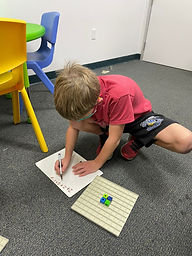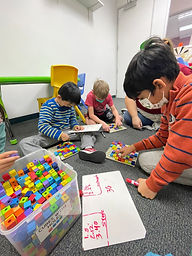Ms. Briana

Target 1​

Lesson Type:

New

Measurment

:

Techniques and Formulas

Find the perimeter of a given shape.

1:

Define perimeter.

2:

Understand that perimeter is the distance around a figure.

3:

Understand that perimeter can be found by adding the lengths of the sides of a figure.

4:

See a link between finding the perimeter of rectangles and multiplication.

3rd

Vocabulary:

Perimeter: the distance around a figure

Activities:

Students were given array boards and cubes and they made squares. Students then counted the sides of all the squares and determined perimeter.

Students tried this will rectangles and then moved onto irregular shapes.

Students creted irregular shapes using the blocks and array boards and switched with their peers caluclating the perimeter of their peers figure.

Studentes were then given a tree print out they had to calculat the perimeter of.Home Exploration

Guiding Questions:Absent Students:

Blake

Target 2

:

1:

Look at a visual spatial puzzle to analyze the pieces and determine which is the correct piece to choose.

3rd

Vocabulary:

Activities:

Students played Bloxus to pracice thinking through how to use space and fit the modt in it.Home Exploration

Guiding Questions:Target 3

:

Vocabulary:

Activities:Home Exploration